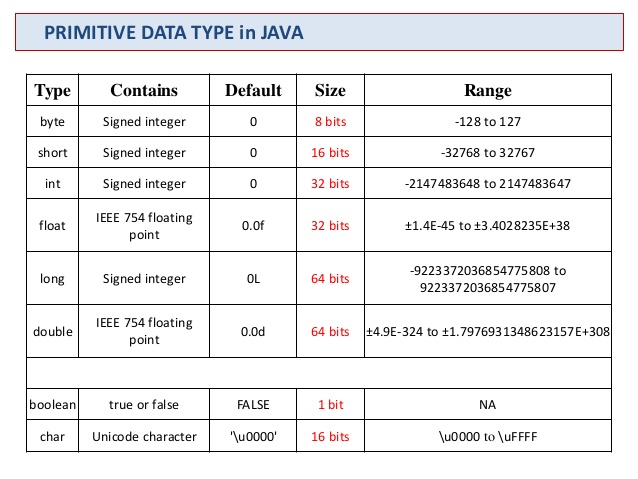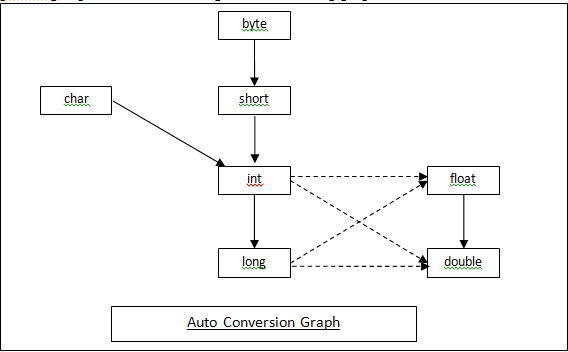#### 自动类型转换

long l = 100;

int i = 200;
long ll = i;int count = 100000000;
int price = 1999;
long totalPrice = count * price;


int count = 100000000;
int price = 1999;
long totalPrice = (long) count * price;


#### 强制类型转换

short s = 199;
int i = s;// 199

double d = 10.24;
long ll = (long) d;// 10


int ii = 300;
byte b = (byte)ii;


300 已经超出了 byte 类型表示的范围，所以会转换成一个毫无意义的数字。

#### 类型提升

long count = 100000000;
int price = 1999;
long totalPrice = price * count;


price 为 int 型，count 为 long 型，运算结果为 long 型，运算结果正常，没有出现溢出的情况。